Mixtures + percentages - math problems

1. Copper sulphate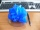How much g of water do we have to add to 240 g of an 84% CuSO4 solution to produce a 60% solution? (Express the mass of crystalline CuSO4 in the original solution and in the resulting solution and compare them. )
2. Vinegar 2How many percentage of getvinegar solution, if we mix to 3.5 liters of 5.8% and 5 liters of 7.6% vinegar?
3. AlcoholHow many 55% alcohol we need to pour into 14 liters 75% alcohol to get p3% of the alcohol? How many 65% alcohol we get?
4. Sea waterSeawater contains about 4.3% salt. How many dm3 of distilled water we must pour into 5 dm3 of sea water to get water with 1.8% salt?
5. Sea waterMixing 34 kg of sea water with 34 kg rainwater is created water containing 3.4% salt. How many percent sea water contains salt?
6. Acid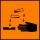The barrel is 199 litres of 38% acid. How many percent acid occurs when 61 litres revoke and replace it with the same amount of water?
7. Vinegar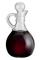We must dilute 16 liters of 8.8% aqueous vinegar to 4.1% one. How much water is necessary to add?
8. Peroxide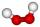How much distilled water (in liters) must pharmacists pour into 300 ml of 23.6% solution of hydrogen peroxide to get 2.7% solution to gargle?
9. Fertilizer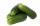Mr. Gherkin use for fertilization 8% solution of fertilizer. 4 liters of it still left. How much water must be added to the solution to make only 4% solution?
10. Acids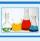70% acid was make from same acid of two different concentrations. Amount weaker acid to the stronger acid is in ratio 2:1. What was the concentration of the weaker acid, if stronger had 91% concentration?
11. PharmacyAt the pharmacy are in one container 20% solution in the second 50% solution of disinfectant. They need to prepare 4 L of 48-percent solution. What amount of solution from each container is needed to mix?
12. Metal alloy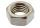What is the ratio of metals in the alloy that is in the 50 tonnes of steel to 30 kg nickel?
13. SpiritFrom 55% and 80% spirit we would like to produce 0.2 kg of 60% spirit. How many of them we must use in a solution?
14. Bronze, tin and copper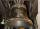Bronze is an alloy of tin and copper. An alloy of 10% tin and 90% copper is Gunmetal. If it contains 20% tin and 80% copper, it is bell metal. How many tons of molten bell metal and how many tons of copper is needed to make 100 tons of Gunmetal?
15. Disinfecting solutionHow much distilled water is necessary to pour into 500 ml of 33% hydrogen peroxide solution to obtain 3% disinfecting solution?
16. Vinegar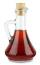What percentage vinegar we get if we mix 1 if dm³ eight percent vinegar with 1.5 dm³ six percent vinegar?
17. SolutionWhat amount of 80% solution and what amount of water is needed to mix in order to obtain two liters of 60% solution of this mixture?
18. Concentration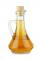How 0.5 liters of 8% vinegar diluted to a concentration of 20 hundredths % of vinegar? How many liters of water must be pour?
19. SaltSea water containing 5% salt. How many kg of fresh water necessary to pour into 40 kg of sea water so that the salinity fell to 2%?
20. ConcentrationHow many grams of 65% and 50% acid is to be mixed to give 240 g acid with a concentration of 60%?

Do you have an interesting mathematical word problem that you can't solve it? Enter it, and we can try to solve it.

To this e-mail address, we will reply solution; solved examples are also published here. Please enter e-mail correctly and check whether you don't have a full mailbox.

Our percentage calculator will help you quickly calculate various typical tasks with percentages.